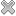Courses / Module

Toggle PrintSIGNALS & SYSTEMS

Module code: EE301FZ
Credits: 5
Semester: 1
Department: INTERNATIONAL ENGINEERING COLLEGE
International:Overview

 Introduction:
Signals, Systems, Linear Systems, Time-Invariant Systems, Continuous-time signals and systems, Discrete-time signals and systems, Fourier Series representation of signals and systems.
 Continuous-time signals and systems:
The impulse function, properties of the impulse function, Fourier transform of the impulse function, impulse response and frequency response of continuous-time systems, convolution, the sifting integral, the Laplace transform, pole-zero models.
 Discrete-time signals and systems:
Sampling, uniform and non-uniform quantization, Linear difference equations, frequency response of discrete-time systems, discrete-time convolution and unit sample response, sampled bandpass signals. Intro to digital filters.
 The Z-transform: Convergence of the ZT, properties of the ZT, Applications of the ZT.
 The Fourier transform (FT):
The discrete-time FT (DTFT), properties of the DTFT, Parseval's Theorem, Sampled signal spectrum, Repeat spectra, Alias, Periodogram.
 Random Signals:
Types of noise, Frequency-response estimation using noise. Random variables, Probability Density Function (PDF), Random processes. Spectral estimation. The periodogram. Bartlett's method.Learning OutcomesTeaching & Learning methodsAssessmentRepeat optionsTimetable